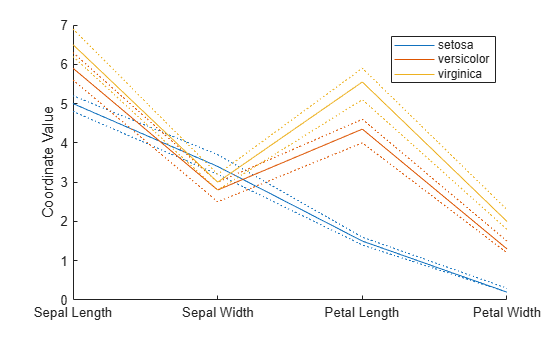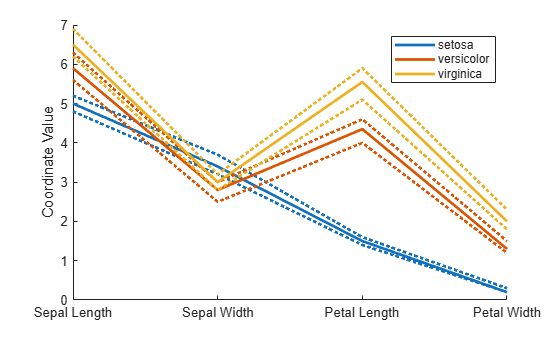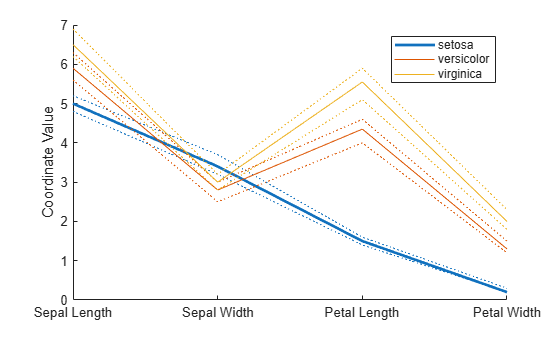# parallelcoords

Parallel coordinates plot

## Syntax

``parallelcoords(x)``
``parallelcoords(x,Name,Value)``
``parallelcoords(ax,___)``
``h = parallelcoords(___)``

## Description

````parallelcoords(x)` creates a parallel coordinates plot of the multivariate data in the matrix `x`. Use a parallel coordinates plot to visualize high dimensional data, where each observation is represented by the sequence of its coordinate values plotted against their coordinate indices.```

example

````parallelcoords(x,Name,Value)` creates a parallel coordinates plot with additional options specified by one or more `Name,Value` pair arguments. For example, you can standardize the data in `x` or label the coordinate tick marks along the horizontal axis of the plot.```
````parallelcoords(ax,___)` creates a parallel coordinates plot using the axes specified by the axes graphic object `ax`, using any of the previous syntaxes.```

example

````h = parallelcoords(___)` returns a column vector of handles to the `Line` objects created by `parallelcoords`, with one handle for each row of `x`.```

## Examples

collapse all

Load the Fisher iris sample data.

`load fisheriris`

The data contains four measurements (sepal length, sepal width, petal length, and petal width) from three species of iris flowers. The matrix `meas` contains all four measurements for each of 150 flowers. The cell array `species` contains the species name for each of the 150 flowers.

Create a cell array that contains the name of each measurement variable in the sample data.

`labels = {'Sepal Length','Sepal Width','Petal Length','Petal Width'};`

Create a parallel coordinate plot using the measurement data in `meas`. Use a different color for each group as identified in `species`, and label the horizontal axis using the variable names.

`parallelcoords(meas,'Group',species,'Labels',labels)`The resulting plot contains one line for each observation (flower). The color of each line indicates the flower species.

Load the Fisher iris sample data.

`load fisheriris`

The data contains four measurements (sepal length, sepal width, petal length, and petal width) from three species of iris flowers. The matrix `meas` contains all four measurements for each of 150 flowers. The cell array `species` contains the species name for each of the 150 flowers.

Create a cell array that contains the name of each measurement variable in the sample data.

`labels = {'Sepal Length','Sepal Width','Petal Length','Petal Width'};`

Create a parallel coordinates plot using the measurement data in `meas`. Plot only the median, 25 percent, and 75 percent quartile values for each group identified in `species`. Label the horizontal axis using the variable names.

```parallelcoords(meas,'group',species,'labels',labels,... 'quantile',.25)```The plot shows the median values for each group as a solid line and the quartile values as dotted lines of the same color. For example, the solid blue line shows the median value measured for each variable on `setosa` irises. The dotted blue line below the solid blue line shows the 25th percentile of measurements for each variable on `setosa` irises. The dotted blue line above the solid blue line shows the 75th percentile of measurements for each variable on `setosa` irises.

Load the Fisher iris sample data.

`load fisheriris`

The data contains four measurements (sepal length, sepal width, petal length, and petal width) from three species of iris flowers. The matrix `meas` contains all four measurements for each of 150 flowers. The cell array `species` contains the species name for each of the 150 flowers.

Create a cell array that contains the name of each measurement variable in the sample data.

`labels = {'Sepal Length','Sepal Width','Petal Length','Petal Width'};`

Create a parallel coordinates plot using the measurement data in `meas`. Plot only the median, 25 percent, and 75 percent quartile values for each group identified in `species`. Label the horizontal axis using the variable names. Set the line width to 2.

```parallelcoords(meas,'group',species,'labels',labels,... 'quantile',.25,'LineWidth',2)```Specifying `'LineWidth'` in this way sets the width of every line in the plot to 2.

Recreate the parallel coordinates plot, but this time, use handles to increase the width of only the line representing the median value for each measurement made on irises in the `setosa` group.

```h = parallelcoords(meas,'group',species,'labels',labels,... 'quantile',.25)``````h = 9x1 Line array: Line (median) Line (lower quantile) Line (upper quantile) Line (median) Line (lower quantile) Line (upper quantile) Line (median) Line (lower quantile) Line (upper quantile) ```

The returned column vector `h` contains handles that correspond to each line object created by `parallelcoords`. For example, h(1) corresponds to the median line for the first grouping variable (`setosa`).

Use dot notation to increase the width of the line showing the median value for each measurement made on irises in the `setosa` group.

`h(1).LineWidth = 2;`## Input Arguments

collapse all

Multivariate input data, specified as an n-by-p matrix of numeric values. n is the number of rows of `x`, and each row corresponds to an observation in `x`. p is the number of columns in `x`, and each column corresponds to a variable in `x`.

`parallelcoords` treats `NaN` values in `x` as missing values and does not plot those coordinate values.

Data Types: `single` | `double`

Axes for plot, specified as an axes graphic object. If you do not specify `ax`, then `parallelcoords` creates the plot using the current axis. For more information on creating an axes graphic object, see `axes` and Axes Properties.

### Name-Value Pair Arguments

Specify optional comma-separated pairs of `Name,Value` arguments. `Name` is the argument name and `Value` is the corresponding value. `Name` must appear inside quotes. You can specify several name and value pair arguments in any order as `Name1,Value1,...,NameN,ValueN`.

Example: `'Group',species,'Quantile',.25` plots the median, 25 percent, and 75 percent quartile values for the input data, using a different color for each group identified in the variable `species`.

Grouping variable for input data, specified as the comma-separated pair consisting of `'Group'` and a numeric array containing a group index for each observation. Alternatively, the array can be a categorical variable, character matrix, string array, or cell array containing a group name for each observation.

Data Types: `single` | `double` | `categorical` | `char` | `string` | `cell`

Horizontal axis labels, specified as the comma-separated pair consisting of `'Labels'` and a character array, string array, or cell array containing the label names.

Example: `'Labels',{'Sepal Width','Sepal Length'}`

Data Types: `char` | `string` | `cell`

Quantiles of input data to plot, specified as the comma-separated pair consisting of `'Quantile'` and a numeric value in the range (0,1). If you specify a value alpha for `'Quantile'`, then `parallelcoords` plots only the median, alpha, and 1 – alpha quantiles for each of the variables (columns) in `x`.

The quantile plot option provides a useful summary of the data when `x` contains many observations.

Example: `'Quantile',.25`

Data Types: `single` | `double`

Method to standardize input data, specified as the comma-separated pair consisting of `'Standardize'` and one of the following.

 `'on'` Scale each column of `x` to have a mean equal to 0 and a standard deviation equal to 1 before plotting. `'PCA'` Create plot from the principal component scores of `x`, in order of decreasing eigenvalues. `parallelcoords` removes rows of `x` containing missing values (`NaN`) for PCA standardization. `'PCAStd'` Create plot using the standardized principal component scores.

Example: `'Standardize','on'`

Tips
• You can modify certain aspects of the plot lines by specifying a property name and value for any of the properties listed in Line Properties. However, this approach applies the modification to all the lines in the plot. To modify only certain plot lines, use the syntax that returns graphics handles and use dot notation to adjust each line property individually. For an illustration, see Adjust Line Properties in Parallel Coordinates Plot.

## Output Arguments

collapse all

Graphic handles for line objects, returned as a vector of `Line` graphic handles. Graphic handles are unique identifiers that you can use to query and modify the properties of a specific line on the plot. To view and set properties of line objects, use dot notation. For information on using dot notation, seeAccess Property Values. For information on the `Line` properties that you can set, see Line Properties.

If you use the `'Quantile'` name-value pair argument, then `h` contains one handle for each of the three lines objects created. If you use both the `'Quantile'` and the `'Group'` name-value pair arguments, then `h` contains three handles for each group.

## Alternative Functionality

Alternatively, you can create a `ParallelCoordinatesPlot` object by using the `parallelplot` function.

• Unlike the `parallelcoords` function, `parallelplot` allows you to plot tabular data that includes categorical variables.

• `parallelplot` does not support the plotting of quantiles for numeric data. However, the `ParallelCoordinatesPlot` object contains the `DataNormalization` property, which provides several data normalization methods for coordinates with numeric values.

To control the appearance and behavior of the object, change the ParallelCoordinatesPlot Properties.